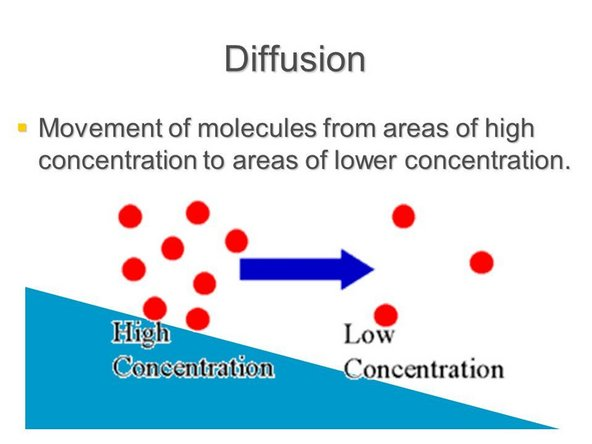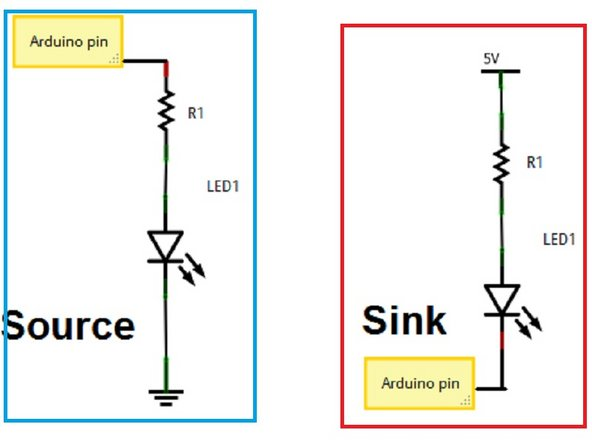1. ## Step 1 The Movement of Current• This is meant to teach you about a concept with software, and requires no hardware at all.

• Current, the flow of electrons, flows from high concentration to low concentration. That means that if one position in a circuit has five volts and another section has zero volts, the current will flow from the five volt section to the zero volt section.

2. ## Step 2 Sourcing Current• While talking about how current moves, it is relative to the position of the Arduino / processing machine.

• When an Arduino is sourcing current, that means that the current is coming from Arduino itself. The Arduino can only push a certain amount of current and can be a limiting factor because some sensors require more current than the Arduino can push.

• When an Arduino is sourcing current, that means outputting the "max" value on any actuator, it will be running at maximum strength because the potential difference between the Arduino and the actuator will be at its highest. It will be outputting "X" volts to an area with 0 volts.

3. ## Step 3 Sinking Current

• While sinking current, the current is instead flowing from an external power source into the Arduino itself. This is the usually the layout for circuits with larger machines because they require more current, and the Arduino itself cannot output the needed current for them to run.

• When writing software for a circuit that is sinking current, outputting a value of 0 from the Arduino will have the actuator operate at full power. That is because the potential difference between the processor and the actuator will be at it's highest.

• Also, the maximum current that an Arduino can sink is significantly higher than any current that it can output. The max output caps out around 5 V while the input it can handle is around 12 V.

Finish Line

### Author

#### Jonathan Lam

Member since: 07/05/2018

97 Reputation

3 Guides authored